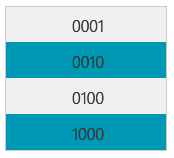#### ByJose Cherian

Mar 30, 2019Handling numbers as text for the first time in Tableau  is a frustrating experience. Depending on your requirements, you might have to add /remove  trailing/ leading zeros, modify number of decimal places etc. This post is an attempt to address the  most common scenarios.

Note:  If the final result required is a number, you can simply convert the string to number and apply Tableau’s built in format rules. This post assumes that the required result is still a string.

This is a common scenario for formatted IDs which are padded with x number of zeros.

This can be achieved using below calculation. It looks for strings starting with 1 to 5 zeros and replaces it with an empty string.

`REGEXP_REPLACE([Original String],"^0{1,5}","")`

2. Removing Trailing Zeros

This scenario normally happens when you want to remove the extra zeros after the decimal point.

This can be achieved using below calculation. It will look  for strings ending with 1 to 3 zeros and an optional decimal point, then replace it with an empty string.

`REGEXP_REPLACE([Original String],"\.?0{1,3}\$","")`

This is the reverse of scenario 1  where you want to pad  a list of IDs with zeros to make it 6 digits.

It is done using below simple calculation

`RIGHT("000000"+[Original String],6)`

This is the reverse of scenario 2 and is slightly more difficult than the other cases. If you are handling it as a number, you can simply define the number of decimal places, but what if you have to handle it as string?

```SPLIT([Original String],".",1)